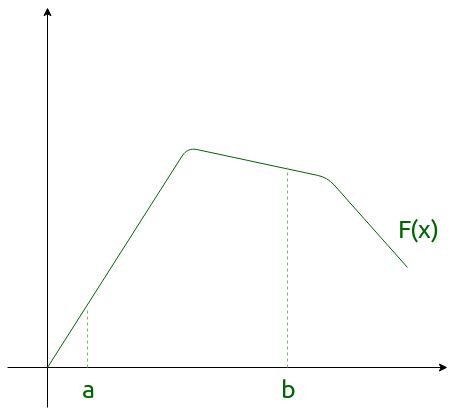# Definite Integral | Mathematics

• Last Updated : 20 May, 2019

Definite integrals are the extension after indefinite integrals, definite integrals have limits [a, b]. It gives the area of a curve bounded between given limits., It denotes the area of curve F(x) bounded between a and b, where a is the lower limit and b is the upper limit.Attention reader! Don’t stop learning now. Get hold of all the important CS Theory concepts for SDE interviews with the CS Theory Course at a student-friendly price and become industry ready.

Note: If f is a continuous function defined on the closed interval [a, b] and F be an anti derivative of f. ThenHere, the function f needs to be well defined and continuous in [a, b].

Example:Solution:### Properties of definite integrals –

1.2.3.4.5.6.7.8.These properties can be used directly to find the value of particular definite integral and also interchange to other forms if required.

My Personal Notes arrow_drop_up